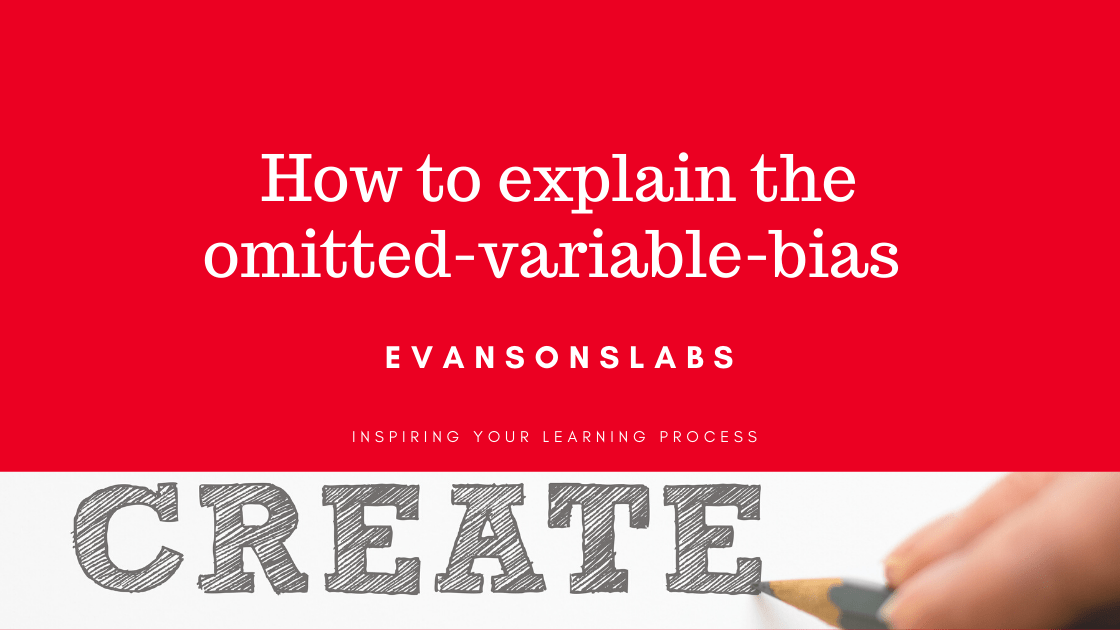# How to explain the Omitted Variable Bias

In regression analysis, the omitted-variable-bias is the error that is incurred on partial-effects-coefficients of other explanatory variables in a restricted regression model. Assume a simple regression model, where Variable $y_i$ is explained by the Variable $x_{1i}$ and the error term $e_i$ for $i=[1,2,3, … , n]$ observations:

y_i=\beta_0+\beta_1\cdot x_{1i}+ e_i \,, \forall i=[ 1, 2, 3, …,n]

Then consider the hypothesis, that a Variable $x_{2i}$ explains the dependent variable $y_i$ and can be depicted by the following extended regression model:

y_i=\tilde\beta_0 + \tilde\beta_1 \cdot x_{1i} + \tilde\beta_2 \cdot x_{2i} + v_i

Setting both equations equal and solving for the error term of the simple:

e_i=(\tilde\beta_0-\beta_0)+(\tilde\beta_1-\beta_1)\cdot x_{1i} +\tilde\beta_2 \cdot x_{2i} + v_i

The error term $e_i$ in the simple regression model includes the deviation of $\tilde\beta_0$ and $\tilde\beta_2$ of the extended regression model from the former coefficients. The partial effects of the omitted variables $\tilde\beta_2$ and the error term of the extended regression model $v_i$ are also included in the error term of the simple regression model. Two factors play a role in the quantification of the omitted-variable-bias:

1. Partial effects of the omitted-variable on the explained variable.
2. Correlation and Covariance of the omitted variable with the rest of the explanatory variables

## Partial effects of Omitted Variable and Correlation with Other Explanatory Variables

Two outcomes are possible: either there is no bias or there is a positive bias or negative bias on the partial effects of other explanatory variables in the restricted model.

### A. No Bias Scenario

If the omitted-variable has zero partial effects in the unrestricted model or zero correlation/covariance (independence between explanatory variables) there is no bias incurred on other partial effects in the restricted model.

### B. Negative Bias Scenario

Negative (positive) partial effects of omitted-variable and positive (negative) correlation with other explanatory variables simultaneously leads to a negative bias on the partial effects of other partial effects of explanatory variables in the restricted model. In this case the signs are in opposite terms (+ and – ).

### C. Positive Bias Scenario

Positive partial effects of omitted-variable and positive correlation with other explanatory variables simultaneously lead to a negative bias on the partial effects of other partial effects of explanatory variables in the restricted model. Similarly, if we simultaneously have negative signs. In this case we have two possible constellations ( + and +) or (- and – ).

Share